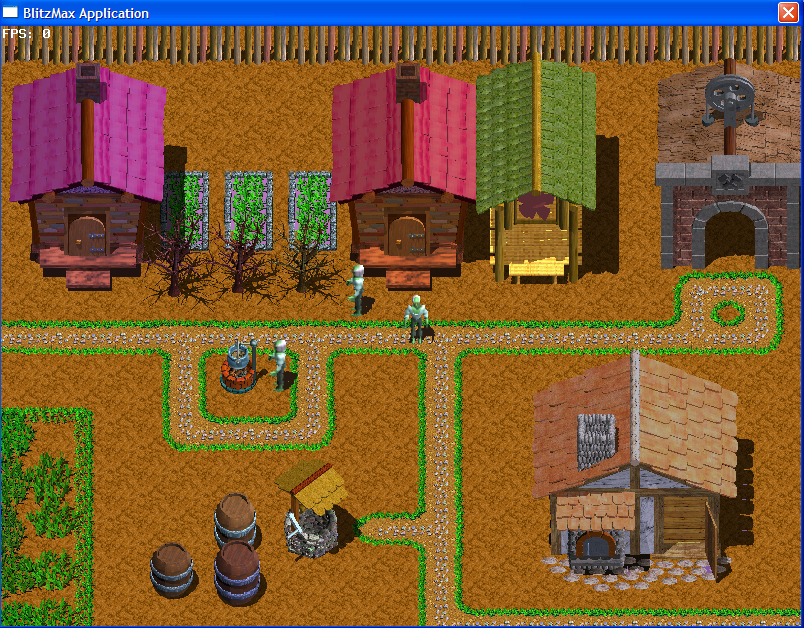-=+=- -=+=- -=+=- -=+=- -=+=- -=+=- -=+=- -=+=- -=+=- -=+=- -=+=- -=+=- -=+=- -=+=- -=+=- -=+=- -=+=- -=+=- -=+=- -=+=- -=+=- -=+=- -=+=- -=+=- -=+=- -=+=- -=+=- -=+=- -=+=- -=+=- (c) WidthPadding Industries 1987 0|623|0 -=+=- -=+=- -=+=- -=+=- -=+=- -=+=- -=+=- -=+=- -=+=- -=+=- -=+=- -=+=- -=+=- -=+=- -=+=- -=+=- -=+=- -=+=- -=+=- -=+=- -=+=- -=+=- -=+=- -=+=- -=+=- -=+=- -=+=- -=+=- -=+=- -=+=-
SoCoder -> Showcase Home -> Role Playing

curtasticCreated : 19 July 2007
Edited : 18 August 2017
System : Windows
Language : Blitz Max

### Eat Brains

Fantasy RPG

rpg.exe (43 MB)
ScreenshotsYou are a zombie and you have the power to change form. Press tab.
Eat brains of monsters to capture their essence and then you can morph into them.
Level up and learn new skills.
Capture the swamp monster so you can swim across rivers.
Capture the skeleton to walk on lava.

## Latest Update

Beta version uploaded on July 19, 2007
Updated on August 2, 2007

Credits:

All graphics by Reiner "Tiles" Prokein "https://www.reinerstileset.de"

Town music by Scott Rowe

Sound effects by "https://freesoundfiles.tintagel.net/Audio/"

Sound effects by "https://www.grsites.com"

2D Engine by Mark Sibly. "https://www.blitzmax.com"

Exe compression by UPX "https://upx.sourceforge.net"